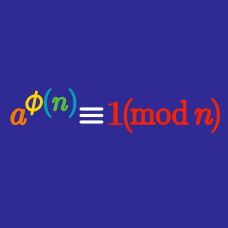Number Theory

# Euler's Totient Function

Given that $30=2\times3\times5$, what is the value of the Euler totient function of 30, i.e. what is $\phi(30)$?

Given that the only prime factors of a certain positive integer $n$ are 2 and 3, find (in terms of $n$) the number of positive integers less than or equal to $n$ that are coprime to $n$.

$\dfrac1{24} , \dfrac{2}{24}, \dfrac{3}{24}, \ldots , \dfrac{23}{24}, \dfrac{24}{24}$

How many of the fractions above cannot be reduced?

$\dfrac1{32} , \dfrac{2}{32}, \dfrac{3}{32}, \ldots , \dfrac{31}{32}, \dfrac{32}{32}$

Which of the following is true regarding the 32 fractions above?

Statement 1: The number of fractions that cannot be reduced is less than the number of fractions that can be reduced.

Statement 2: The number of fractions that cannot be reduced is equal to the number of fractions that can be reduced.

Statement 3: The number of fractions that cannot be reduced is greater than the number of fractions that can be reduced.

Let $A$ denote the number of positive integers less than or equal to 11 that are coprime with 11.

Let $B$ denote the number of positive integers less than or equal to 12 that are coprime with 12.

Which one is larger, $A$ or $B$?

×